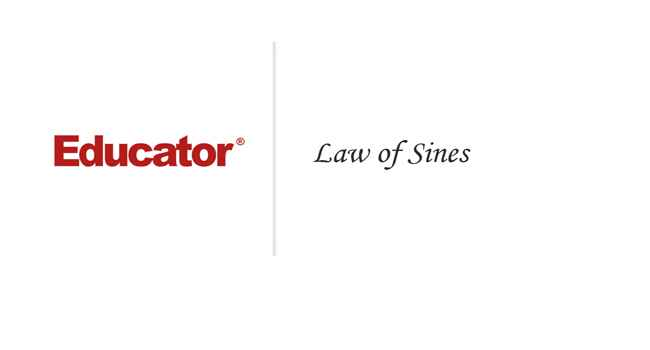Professor Murray

Law of Sines

Slide Duration:

Section 1: Trigonometric Functions
Angles

39m 5s

Intro
0:00
Degrees
0:22
Circle is 360 Degrees
0:48
Splitting a Circle
1:13
2:08
2:31
2:52
Half-Circle and Right Angle
4:00
6:24
6:52
Coterminal, Complementary, Supplementary Angles
7:23
Coterminal Angles
7:30
Complementary Angles
9:40
Supplementary Angles
10:08
Example 1: Dividing a Circle
10:38
Example 2: Converting Between Degrees and Radians
11:56
Example 3: Quadrants and Coterminal Angles
14:18
Extra Example 1: Common Angle Conversions
-1
Extra Example 2: Quadrants and Coterminal Angles
-2
Sine and Cosine Functions

43m 16s

Intro
0:00
Sine and Cosine
0:15
Unit Circle
0:22
Coordinates on Unit Circle
1:03
Right Triangles
1:52
2:25
Master Right Triangle Formula: SOHCAHTOA
2:48
Odd Functions, Even Functions
4:40
Example: Odd Function
4:56
Example: Even Function
7:30
Example 1: Sine and Cosine
10:27
Example 2: Graphing Sine and Cosine Functions
14:39
Example 3: Right Triangle
21:40
Example 4: Odd, Even, or Neither
26:01
Extra Example 1: Right Triangle
-1
Extra Example 2: Graphing Sine and Cosine Functions
-2
Sine and Cosine Values of Special Angles

33m 5s

Intro
0:00
45-45-90 Triangle and 30-60-90 Triangle
0:08
45-45-90 Triangle
0:21
30-60-90 Triangle
2:06
Mnemonic: All Students Take Calculus (ASTC)
5:21
Using the Unit Circle
5:59
New Angles
6:21
9:43
Mnemonic: All Students Take Calculus
10:13
13:11
16:48
Example 3: All Angles and Quadrants
20:21
Extra Example 1: Convert, Quadrant, Sine/Cosine
-1
Extra Example 2: All Angles and Quadrants
-2
Modified Sine Waves: Asin(Bx+C)+D and Acos(Bx+C)+D

52m 3s

Intro
0:00
Amplitude and Period of a Sine Wave
0:38
Sine Wave Graph
0:58
Amplitude: Distance from Middle to Peak
1:18
Peak: Distance from Peak to Peak
2:41
Phase Shift and Vertical Shift
4:13
Phase Shift: Distance Shifted Horizontally
4:16
Vertical Shift: Distance Shifted Vertically
6:48
Example 1: Amplitude/Period/Phase and Vertical Shift
8:04
Example 2: Amplitude/Period/Phase and Vertical Shift
17:39
Example 3: Find Sine Wave Given Attributes
25:23
Extra Example 1: Amplitude/Period/Phase and Vertical Shift
-1
Extra Example 2: Find Cosine Wave Given Attributes
-2
Tangent and Cotangent Functions

36m 4s

Intro
0:00
Tangent and Cotangent Definitions
0:21
Tangent Definition
0:25
Cotangent Definition
0:47
Master Formula: SOHCAHTOA
1:01
Mnemonic
1:16
Tangent and Cotangent Values
2:29
Remember Common Values of Sine and Cosine
2:46
90 Degrees Undefined
4:36
Slope and Menmonic: ASTC
5:47
Uses of Tangent
5:54
Example: Tangent of Angle is Slope
6:09
7:49
Example 1: Graph Tangent and Cotangent Functions
10:42
Example 2: Tangent and Cotangent of Angles
16:09
Example 3: Odd, Even, or Neither
18:56
Extra Example 1: Tangent and Cotangent of Angles
-1
Extra Example 2: Tangent and Cotangent of Angles
-2
Secant and Cosecant Functions

27m 18s

Intro
0:00
Secant and Cosecant Definitions
0:17
Secant Definition
0:18
Cosecant Definition
0:33
Example 1: Graph Secant Function
0:48
Example 2: Values of Secant and Cosecant
6:49
Example 3: Odd, Even, or Neither
12:49
Extra Example 1: Graph of Cosecant Function
-1
Extra Example 2: Values of Secant and Cosecant
-2
Inverse Trigonometric Functions

32m 58s

Intro
0:00
Arcsine Function
0:24
Restrictions between -1 and 1
0:43
Arcsine Notation
1:26
Arccosine Function
3:07
Restrictions between -1 and 1
3:36
Cosine Notation
3:53
Arctangent Function
4:30
Between -Pi/2 and Pi/2
4:44
Tangent Notation
5:02
Example 1: Domain/Range/Graph of Arcsine
5:45
Example 2: Arcsin/Arccos/Arctan Values
10:46
Example 3: Domain/Range/Graph of Arctangent
17:14
Extra Example 1: Domain/Range/Graph of Arccosine
-1
Extra Example 2: Arcsin/Arccos/Arctan Values
-2
Computations of Inverse Trigonometric Functions

31m 8s

Intro
0:00
Inverse Trigonometric Function Domains and Ranges
0:31
Arcsine
0:41
Arccosine
1:14
Arctangent
1:41
Example 1: Arcsines of Common Values
2:44
Example 2: Odd, Even, or Neither
5:57
Example 3: Arccosines of Common Values
12:24
Extra Example 1: Arctangents of Common Values
-1
Extra Example 2: Arcsin/Arccos/Arctan Values
-2
Section 2: Trigonometric Identities
Pythagorean Identity

19m 11s

Intro
0:00
Pythagorean Identity
0:17
Pythagorean Triangle
0:27
Pythagorean Identity
0:45
Example 1: Use Pythagorean Theorem to Prove Pythagorean Identity
1:14
Example 2: Find Angle Given Cosine and Quadrant
4:18
Example 3: Verify Trigonometric Identity
8:00
Extra Example 1: Use Pythagorean Identity to Prove Pythagorean Theorem
-1
Extra Example 2: Find Angle Given Cosine and Quadrant
-2
Identity Tan(squared)x+1=Sec(squared)x

23m 16s

Intro
0:00
Main Formulas
0:19
Companion to Pythagorean Identity
0:27
For Cotangents and Cosecants
0:52
How to Remember
0:58
Example 1: Prove the Identity
1:40
Example 2: Given Tan Find Sec
3:42
Example 3: Prove the Identity
7:45
Extra Example 1: Prove the Identity
-1
Extra Example 2: Given Sec Find Tan
-2

52m 52s

Intro
0:00
0:09
How to Remember
0:48
Cofunction Identities
1:31
How to Remember Graphically
1:44
Where to Use Cofunction Identities
2:52
Example 1: Derive the Formula for cos(A-B)
3:08
Example 2: Use Addition and Subtraction Formulas
16:03
Example 3: Use Addition and Subtraction Formulas to Prove Identity
25:11
Extra Example 1: Use cos(A-B) and Cofunction Identities
-1
Extra Example 2: Convert to Radians and use Formulas
-2
Double Angle Formulas

29m 5s

Intro
0:00
Main Formula
0:07
How to Remember from Addition Formula
0:18
Two Other Forms
1:35
Example 1: Find Sine and Cosine of Angle using Double Angle
3:16
Example 2: Prove Trigonometric Identity using Double Angle
9:37
Example 3: Use Addition and Subtraction Formulas
12:38
Extra Example 1: Find Sine and Cosine of Angle using Double Angle
-1
Extra Example 2: Prove Trigonometric Identity using Double Angle
-2
Half-Angle Formulas

43m 55s

Intro
0:00
Main Formulas
0:09
Confusing Part
0:34
Example 1: Find Sine and Cosine of Angle using Half-Angle
0:54
Example 2: Prove Trigonometric Identity using Half-Angle
11:51
Example 3: Prove the Half-Angle Formula for Tangents
18:39
Extra Example 1: Find Sine and Cosine of Angle using Half-Angle
-1
Extra Example 2: Prove Trigonometric Identity using Half-Angle
-2
Section 3: Applications of Trigonometry
Trigonometry in Right Angles

25m 43s

Intro
0:00
Master Formula for Right Angles
0:11
SOHCAHTOA
0:15
Only for Right Triangles
1:26
Example 1: Find All Angles in a Triangle
2:19
Example 2: Find Lengths of All Sides of Triangle
7:39
Example 3: Find All Angles in a Triangle
11:00
Extra Example 1: Find All Angles in a Triangle
-1
Extra Example 2: Find Lengths of All Sides of Triangle
-2
Law of Sines

56m 40s

Intro
0:00
Law of Sines Formula
0:18
SOHCAHTOA
0:27
Any Triangle
0:59
Graphical Representation
1:25
Solving Triangle Completely
2:37
When to Use Law of Sines
2:55
ASA, SAA, SSA, AAA
2:59
SAS, SSS for Law of Cosines
7:11
Example 1: How Many Triangles Satisfy Conditions, Solve Completely
8:44
Example 2: How Many Triangles Satisfy Conditions, Solve Completely
15:30
Example 3: How Many Triangles Satisfy Conditions, Solve Completely
28:32
Extra Example 1: How Many Triangles Satisfy Conditions, Solve Completely
-1
Extra Example 2: How Many Triangles Satisfy Conditions, Solve Completely
-2
Law of Cosines

49m 5s

Intro
0:00
Law of Cosines Formula
0:23
Graphical Representation
0:34
Relates Sides to Angles
1:00
Any Triangle
1:20
Generalization of Pythagorean Theorem
1:32
When to Use Law of Cosines
2:26
SAS, SSS
2:30
Heron's Formula
4:49
Semiperimeter S
5:11
Example 1: How Many Triangles Satisfy Conditions, Solve Completely
5:53
Example 2: How Many Triangles Satisfy Conditions, Solve Completely
15:19
Example 3: Find Area of a Triangle Given All Side Lengths
26:33
Extra Example 1: How Many Triangles Satisfy Conditions, Solve Completely
-1
Extra Example 2: Length of Third Side and Area of Triangle
-2
Finding the Area of a Triangle

27m 37s

Intro
0:00
Master Right Triangle Formula and Law of Cosines
0:19
SOHCAHTOA
0:27
Law of Cosines
1:23
Heron's Formula
2:22
Semiperimeter S
2:37
Example 1: Area of Triangle with Two Sides and One Angle
3:12
Example 2: Area of Triangle with Three Sides
6:11
Example 3: Area of Triangle with Three Sides, No Heron's Formula
8:50
Extra Example 1: Area of Triangle with Two Sides and One Angle
-1
Extra Example 2: Area of Triangle with Two Sides and One Angle
-2
Word Problems and Applications of Trigonometry

34m 25s

Intro
0:00
Formulas to Remember
0:11
SOHCAHTOA
0:15
Law of Sines
0:55
Law of Cosines
1:48
Heron's Formula
2:46
Example 1: Telephone Pole Height
4:01
Example 2: Bridge Length
7:48
Example 3: Area of Triangular Field
14:20
Extra Example 1: Kite Height
-1
Extra Example 2: Roads to a Town
-2
Vectors

46m 42s

Intro
0:00
Vector Formulas and Concepts
0:12
Vectors as Arrows
0:28
Magnitude
0:38
Direction
0:50
Drawing Vectors
1:16
Uses of Vectors: Velocity, Force
1:37
Vector Magnitude Formula
3:15
Vector Direction Formula
3:28
Vector Components
6:27
Example 1: Magnitude and Direction of Vector
8:00
Example 2: Force to a Box on a Ramp
12:25
Example 3: Plane with Wind
18:30
Extra Example 1: Components of a Vector
-1
Extra Example 2: Ship with a Current
-2
Section 4: Complex Numbers and Polar Coordinates
Polar Coordinates

1h 7m 35s

Intro
0:00
Polar Coordinates vs Rectangular/Cartesian Coordinates
0:12
Rectangular Coordinates, Cartesian Coordinates
0:23
Polar Coordinates
0:59
Converting Between Polar and Rectangular Coordinates
2:06
R
2:16
Theta
2:48
Example 1: Convert Rectangular to Polar Coordinates
6:53
Example 2: Convert Polar to Rectangular Coordinates
17:28
Example 3: Graph the Polar Equation
28:00
Extra Example 1: Convert Polar to Rectangular Coordinates
-1
Extra Example 2: Graph the Polar Equation
-2
Complex Numbers

35m 59s

Intro
0:00
Main Definition
0:07
Number i
0:23
Complex Number Form
0:33
Powers of Imaginary Number i
1:00
Repeating Pattern
1:43
Operations on Complex Numbers
3:30
3:39
Multiplying Complex Numbers
4:39
FOIL Method
5:06
Conjugation
6:29
Dividing Complex Numbers
7:34
Conjugate of Denominator
7:45
Example 1: Solve For Complex Number z
11:02
Example 2: Expand and Simplify
15:34
Example 3: Simplify the Powers of i
17:50
Extra Example 1: Simplify
-1
Extra Example 2: All Complex Numbers Satisfying Equation
-2
Polar Form of Complex Numbers

40m 43s

Intro
0:00
Polar Coordinates
0:49
Rectangular Form
0:52
Polar Form
1:25
R and Theta
1:51
Polar Form Conversion
2:27
R and Theta
2:35
Optimal Values
4:05
Euler's Formula
4:25
Multiplying Two Complex Numbers in Polar Form
6:10
Multiply r's Together and Add Exponents
6:32
Example 1: Convert Rectangular to Polar Form
7:17
Example 2: Convert Polar to Rectangular Form
13:49
Example 3: Multiply Two Complex Numbers
17:28
Extra Example 1: Convert Between Rectangular and Polar Forms
-1
Extra Example 2: Simplify Expression to Polar Form
-2
DeMoivre's Theorem

57m 37s

Intro
0:00
Introduction to DeMoivre's Theorem
0:10
n nth Roots
3:06
DeMoivre's Theorem: Finding nth Roots
3:52
Relation to Unit Circle
6:29
One nth Root for Each Value of k
7:11
Example 1: Convert to Polar Form and Use DeMoivre's Theorem
8:24
Example 2: Find Complex Eighth Roots
15:27
Example 3: Find Complex Roots
27:49
Extra Example 1: Convert to Polar Form and Use DeMoivre's Theorem
-1
Extra Example 2: Find Complex Fourth Roots
-2
Bookmark & Share Embed

## Copy & Paste this embed code into your website’s HTML

Please ensure that your website editor is in text mode when you paste the code.
(In Wordpress, the mode button is on the top right corner.)
×
• - Allow users to view the embedded video in full-size.
Since this lesson is not free, only the preview will appear on your website.

• ## Related Books3 answersLast reply by: Dr. William MurrayFri Jul 22, 2016 1:08 PMPost by Peter Ke on June 22, 2016This is just a curious question. For SSA, you could have no solution, 1 solution, and 2 solution. If the value of sin(theta) is in between -1 and 1 it will have 2 solution. If the value of sin(theta) is less than -1 or greater than 1 there is no solution. Then what value of sin(theta) will produce 1 solution because you did not show that in the video?1 answerLast reply by: Dr. William MurrayThu Jun 5, 2014 11:46 AMPost by Charlie Jiang on June 1, 2014Isn't the law of sines this:a/SinA = b/SinB = c/SinC ?1 answerLast reply by: Dr. William MurrayTue Mar 11, 2014 3:55 PMPost by Heather Magnuson on March 6, 2014There are not any examples of a situation with only one solution.  Would this happen when the triangle is a perfect right triangle?1 answerLast reply by: Dr. William MurrayTue Aug 13, 2013 5:12 PMPost by Taylor Wright on July 19, 2013How do you determine which angle for SSA is going to be the one which could have 2 values?1 answerLast reply by: Dr. William MurraySun Apr 28, 2013 11:18 AMPost by JANE CONNER on February 19, 2012If angle A is obtuse, how can you tell that sine B will be less than 1. Is it because sin b is the supplement of sin A?

### Law of Sines

Main formulas:

• The Law of Sines:
 sinAa = sinBb = sinCc
This holds in any triangle - not just right triangles!
• Use the Law of Sines for triangles described in the following ways:
• ASA always has a unique solution (assuming the angles sum to less than 180° ).
• SAA always has a unique solution (assuming the angles sum to less than 180° ).
• SSA might have no solutions, one solution, or two solutions.
• AAA always has infinitely many solutions (assuming the angles sum to 180° ), but the triangles are all similar.
• (Use the Law of Cosines for SAS and SSS.)

Example 1:

In triangle ABC, angle A measures 50° , angle B measures 60° , and side c has length 4. Determine how many triangles satisfy these conditions and solve the triangle(s) completely.

Example 2:

In triangle ABC, side a has length 3, side b has length 4, and angle A measures 40° . Determine how many triangles satisfy these conditions and solve the triangle(s) completely.

Example 3:

In triangle ABC, side a has length 7, side b has length 12, and angle A measures 45° . Determine how many triangles satisfy these conditions and solve the triangle(s) completely.

Example 4:

In triangle ABC, angle A measures 40° , angle B measures 110° , and side a has length 7. Determine how many triangles satisfy these conditions and solve the triangle(s) completely.

Example 5:

In triangle ABC, side a has length 10, side b has length 8, and angle B measures 20° . Determine how many triangles satisfy these conditions and solve the triangle(s) completely.

### Law of Sines

In triangle ABC, angle A measures 150°, angle C measures 20°, and side a has length 200. Determine how many triangles satisfy these conditions and solve the triangle(s) completely.
• Start by drawing a triangle with all the sides and angles labeled properly
•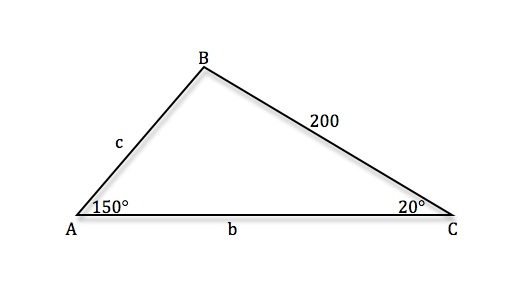• According to the triangle: SAA, and the angles sum to 170° which is less than 180° so there is one unique solution
• Use the Law of Sines: [sinA/a] = [sinB/b] = [sinC/c]
• ∠B = 180° - 150° - 20° = 10°
• [sinA/a] = [sinC/c] ⇒ [(sin150°)/200] = [(sin20°)/c] ⇒ c  sin150° = 200 sin20° ⇒ c = [(200sin20°)/(sin150°)] ⇒ c ≈ 136.8
• [sinA/a] = [sinB/b] ⇒ [(sin150°)/200] = [(sin10°)/b] ⇒ b  sin150° = 200 sin10° ⇒ b = [(200sin10°)/(sin150°)] ⇒ b ≈ 69.5
∠B= 10°, c ≈ 136.8, b ≈ 69.5
In triangle ABC, side a has length 8, side b has length 5, and angle A measures 36°. Determine how many triangles satisfy these conditions and solve the triangle(s) completely.
• Start by drawing a triangle with all the sides and angles labeled properly
•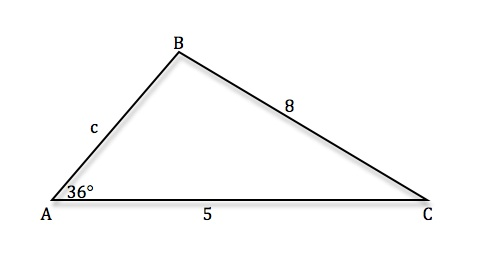• According to the triangle: SSA, might have no solution, one solution, or two solutions
• Use the Law of Sines: [sinA/a] = [sinB/b] = [sinC/c]
• [sinA/a] = [sinB/b] ⇒ [(sin36o)/8] = [sinB/5] ⇒ 5sin36o = 8sinB ⇒ sinB = [(5sin36°)/8] ⇒ sinB ≈ 0.3674 ⇒ B ≈ 21.6°
• ∠ B can also be 180° - 21.6° = 158.4° but C would be 180° - 36° - 158.4° = - 14.4° so this second triangle does not exist
• One solution: ∠ B = 21.6°
• ∠ C = 180° - 36° - 21.6° = 122.4°
• [sinA/a] = [sinC/c] ⇒ [(sin36°)/8] = [(sin122.4°)/c] ⇒ c  sin36° = 8 sin122.4° ⇒ c = [(8sin122.4°)/(sin36°)] ⇒ c ≈ 11.5
∠B = 21.6°, ∠C = 122.4°, c ≈ 11.5
In triangle ABC, angle A measures 43°, angle B measures 75°, and side a has length 6. Determine how many triangles satisfy these conditions and solve the triangle(s) completely.
• Start by drawing a triangle with all the sides and angles labeled properly
•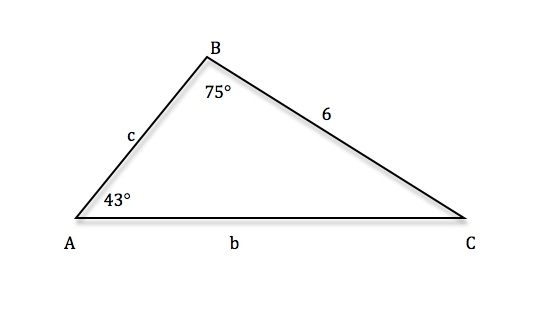• According to the triangle: SAA, and the angles sum to 118° which is less than 180° so there is one unique solution
• Use the Law of Sines: [sinA/a] = [sinB/b] = [sinC/c]
• ∠ C = 180° - 75° - 43° = 62°
• [sinA/a] = [sinB/b] ⇒ [(sin43°)/6] = [(sin75°)/b] ⇒ b  sin43° = 6sin75° ⇒ b = [(6sin75°)/(sin43°)] ⇒ b ≈ 8.5
• [sinA/a] = [sinC/c] ⇒ [(sin43°)/6] = [(sin62°)/c] ⇒ c sin43° = 6sin62° ⇒ c = [(6sin62°)/(sin43°)] ⇒ c ≈ 7.8
∠C = 62°, b ≈ 8.5, c ≈ 7.8
In triangle ABC, side b has length 9, side c has length 11, and angle B measures 65°. Determine how many triangles satisfy these conditions and solve the triangle(s) completely.
• Start by drawing a triangle with all the sides and angles labeled properly
•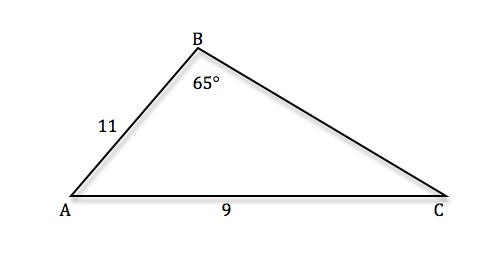• According to the triangle: SSA, might have no solution, one solution, or two solutions
• Use the Law of Sines: [sinA/a] = [sinB/b] = [sinC/c]
• [sinA/a] = [sinC/c] ⇒ [(sin65°)/9] = [sinC/11] ⇒ 9sinC = 11sin65 ⇒ sinC = [(11sin65°)/9] ⇒ sinC ≈ 1.1077
sin C > 1 so no solution
In triangle ABC, angle A measures 24°, angle C measures 54°, and side c has length 2. Determine how many triangles satisfy these conditions and solve the triangle(s) completely.
• Start by drawing a triangle with all the sides and angles labeled properly
•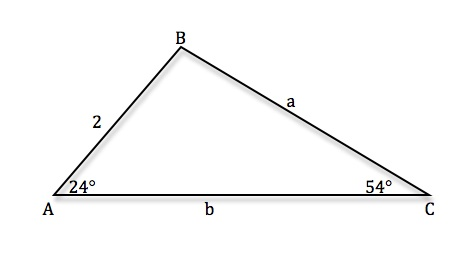• According to the triangle: SAA, and the angles sum to 170° which is less than 180° so there is one unique solution
• Use the Law of Sines: [sinA/a] = [sinB/b] = [sinC/c]
• ∠B = 180° − 54° − 24° = 102°
• [sinA/a] = [sinC/c] ⇒ [(sin24°)/a] = [(sin54°)/2] ⇒ a  sin54° = 2sin24° ⇒ a = [(2sin24°)/(sin54°)] ⇒ a ≈ 1.01
• [sinA/a] = [sinB/b] ⇒ [(sin102°)/b] = [(sin54°)/2] ⇒ 2sin102° = b  sin54° ⇒ b = [(2sin102°)/(sin54°)] ⇒ b ≈ 2.4
∠B = 102°, a ≈ 1.01, b ≈ 2.4
Solve the triangle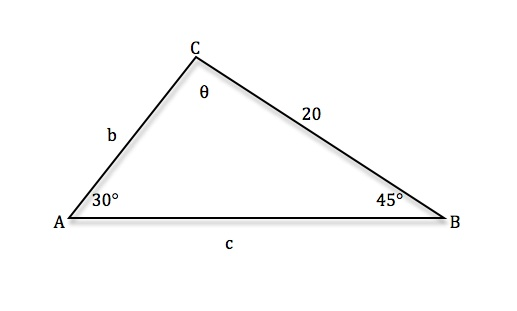• Use the Law of Sines: [sinA/a] = [sinB/b] = [sinC/c]
• ∠C = 180° − 30° − 45° = 105°
• [sinA/a] = [sinB/b] [(sin30°)/20] = [(sin45°)/b] 20sin45° = b  sin30° b = [(20sin45°)/(sin30°)] b ≈ 28.3
• [sinA/a] = [sinC/c] ⇒ [(sin30°)/20] = [(sin105°)/c] ⇒ c  sin30° = 20sin105° ⇒ c = [(20sin105°)/(sin30°)] ⇒ c ≈ 38.6
∠C = 105°, b ≈ 28.3, c ≈ 38.6
Solve the triangle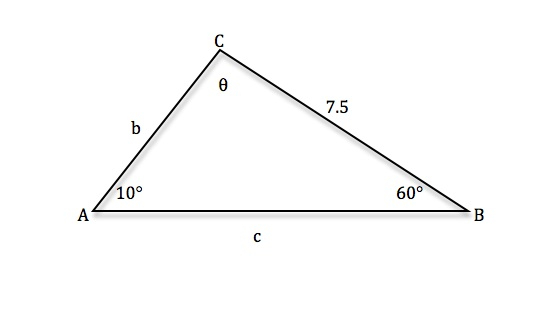• Use the Law of Sines: [sinA/a] = [sinB/b] = [sinC/c]
• ∠C = 180° − 10° − 60° = 110°
• [sinA/a] = [sinB/b] ⇒ [(sin10°)/7.5] = [(sin60°)/b] ⇒ b  sin10° = 7.5sin60° ⇒ b = [(7.5sin60°)/(sin10°)] ⇒ b ≈ 37.4
• [sinA/a] = [sinC/c] ⇒ [(sin10°)/7.5] = [(sin110°)/c] ⇒ c  sin10° = 7.5sin110° ⇒ c = [(7.5sin110°)/(sin10°)] ⇒ c ≈ 40.6
∠C = 110°, b ≈ 37.4, c ≈ 40.6
Solve the triangle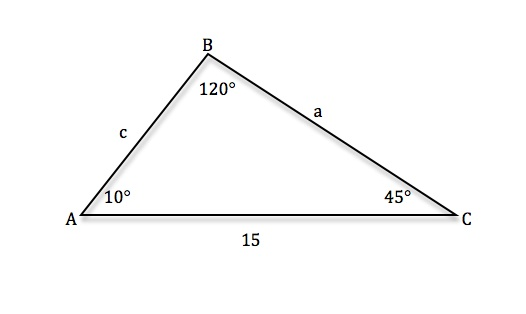• Use the Law of Sines: [sinA/a] = [sinB/b] = [sinC/c]
• ∠A = 180° − 120° − 45° = 15°
• [sinA/a] = [sinB/b] ⇒ [(sin15°)/a] = [(sin120°)/15] ⇒ a  sin120° = 15sin15° ⇒ a = [(15sin15°)/(sin120°)] ⇒ a ≈ 4.5
• [sinB/b] = [sinC/c] ⇒ [(sin120°)/15] = [(sin45°)/c] ⇒ c  sin120° = 15sin45° ⇒ c = [(15sin45°)/(sin120°)] ⇒ c ≈ 12.2
∠A = 15°, a ≈ 4.5, c ≈ 12.2
Solve the triangle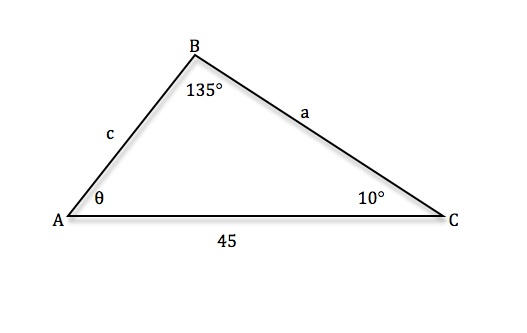• Use the Law of Sines: [sinA/a] = [sinB/b] = [sinC/c]
• ∠A = 180° − 135° − 10° = 35°
• [sinA/a] = [sinB/b] ⇒ [(sin35°)/a] = [(sin135°)/45] ⇒ 45sin35° = a  sin135° ⇒ a = [(45sin35°)/(sin135°)] ⇒ a ≈ 36.5
• [sinB/b] = [sinC/c] ⇒ [(sin135°)/45] = [(sin10°)/c] ⇒ c  sin135° = 45sin10° ⇒ c = [(45sin10°)/(sin135°)] ⇒ c ≈ 11.1
∠A = 35°, a ≈ 36.5, c ≈ 11.1
Solve the triangle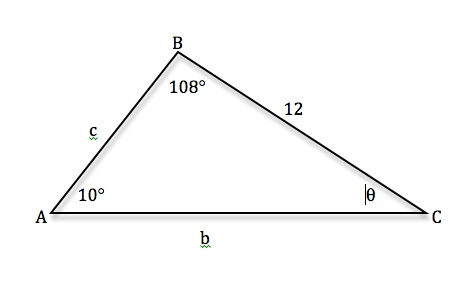• Use the Law of Sines: [sinA/a] = [sinB/b] = [sinC/c]
• ∠C = 180° − 108° − 45° = 47°
• [sinA/a] = [sinB/b] ⇒ [(sin25°)/12] = [(sin108°)/b] ⇒ b  sin25° = 12sin108° ⇒ b = [(12sin108°)/(sin25°)] ⇒ b ≈ 27.0
• [sinA/a] = [sinC/c] ⇒ [(sin25°)/12] = [(sin47°)/c] ⇒ c  sin25° = 12sin47° ⇒ c = [(12sin47°)/(sin25°)] ⇒ c ≈ 20.8
∠C = 47°, b ≈ 27.0, c ≈ 20.8

*These practice questions are only helpful when you work on them offline on a piece of paper and then use the solution steps function to check your answer.

### Law of Sines

Lecture Slides are screen-captured images of important points in the lecture. Students can download and print out these lecture slide images to do practice problems as well as take notes while watching the lecture.

• Intro 0:00
• Law of Sines Formula 0:18
• SOHCAHTOA
• Any Triangle
• Graphical Representation
• Solving Triangle Completely
• When to Use Law of Sines 2:55
• ASA, SAA, SSA, AAA
• SAS, SSS for Law of Cosines
• Example 1: How Many Triangles Satisfy Conditions, Solve Completely 8:44
• Example 2: How Many Triangles Satisfy Conditions, Solve Completely 15:30
• Example 3: How Many Triangles Satisfy Conditions, Solve Completely 28:32
• Extra Example 1: How Many Triangles Satisfy Conditions, Solve Completely
• Extra Example 2: How Many Triangles Satisfy Conditions, Solve Completely

### Transcription: Law of Sines

We are learning about the law of science today, we are trying some more examples of solving triangles completely using the law of science.0000

Remember, that means you are given some data about the triangles, some information about the lengths and some of the sides of the triangle, and the measure of some of the angles.0008

What you have to do, is first of all figure out if there is a triangle that satisfies that data or maybe if there is more than one.0019

And then solve for all the other lengths and sides, and all of the other measures of the angles in the triangle.0027

In this example, we are given a triangle- it looks like we are given two angles and a side, let me draw the triangle.0036

Capital letters for the corners and the sides you will use lower case letters, it has to be opposite of the capital of the same letter.0052

That tells you where the orientation of all the information that you are given, given that (A is 40 degree), angle A=40 degrees, angle B=110 degrees.0063

Certainly the way I drew it is not to scale because 110 would be end up two’s angle, it is bigger than 90 degrees.0074

We are given that (a) has side length=7, side (a) has length=7, I fill in the information that I have.0081

I want to find out first of all, are we going to have a solution here? We are given here a side and then two angles of a triangle.0089

This is (side, angle, angle) the side is not between the angles that is why (side, angle, angle) not (angle, side, angle).0098

The thing you want to check there is whether the angles you have been given are legitimate, did they add up to less than 180 degrees.0109

We talked about that in the beginning of the lecture, when you are given certain pieces of information how do you know if there is one solution or no solutions or two solutions?0121

In (side, angle, angle), you just check whether the angles add up to 180 degrees.0130

In this case, 110 + 40 = 150, that is less than 180 so we have not already exceeded the angle limit for the triangle.0137

In that case, it has a unique solution, it has one solution, that would be exactly one triangle satisfying the data we have been given.0150

That answers the first question, the trickier part is use the law of science to find all the missing quantities here.0166

Let me write down the law of science, sin(A)/a = sin(B)/b = sin(C)/c.0173

What do we know there? We know (A), so we can find its sin very easily, we know (B) and we know (a).0189

We can figure out (C) very quickly because we know two of the angles and it is very easy to find the third one.0200

So, (C) = 110 – 40, sorry it is 180 – the two angles we are given (40+110), so that is 180-150=30 degrees, we can fill that in very quickly, (C)=30 degrees.0207

We got sin(C), we can figure that out quickly, we need to find (b) and (c), we need to use a lot of sin to find them.0230

Sin(A)/a=sin(B)/b and trying to solve for (b) here, I will cross multiply and get b sin(A)=a sin(B) or (b)=a sin(B)/(A).0241

Actually, I’m going to fill in before I cross multiply, I will fill in the quantities that I know, sin(A)=40, (a)=7, (b)=?, (B)=110.0264

Now, I’m going to cross multiply, and I get (b) = (7)sin(110)/sin(40).0281

Remember to set your calculator to degree mode so you do not get strange answers because your calculator is thinking in terms of radians.0291

I will work out (7)sin(110)/sin(40) what I get there is approximately, I’m going to round it to 10.23, so that is the length of side (b).0304

We found all three angles, two of the sides, we just need to find side (c).0327

I’m going to use the law of science. Sin(A)/a=sin(C)/c, angle A=40, a=7, C=30, c= we do not know, that is what we are solving for, so I will cross multiply.0333

I will get c=(7)sin(30)/sin(40), there is actually a common value, I do not what the sin is but it is not really that useful here.0359

Certainly I do not know sin(40), so I’m plugging these numbers into my calculator anyway.0376

(7)sin(30)/sin(40) is approximately equal to 5.45.0381

Let us recap what we needed to do there, we were given two angles and one side of a triangle, I filled in everything I knew, that gave me a (side, angle, angle) configuration.0408

It is not (angle, side, angle) because of the orientation of where everything was, the side was not in between the two angles, it was outside of the two angles.0421

We have (side, angle, angle) and remember from the list at the beginning of the lecture, if you have (side, angle, angle).0432

You just have to check that the angles add up to less than 180 degrees, which they did and then you know you has exactly one solution.0440

Once you determine that, you know you are going to find one solution.0448

It is quick to find the third angle because you know the angles add up to 180 and then you have to find the two missing sides.0453

That takes a little more time but they both come from the law of science.0460

You just write down the law of science, you fill in the information that you do know and then you solve for the missing side.0465

That worked to find both of those missing sides, then we know all three sides of the triangle and all three angles, we completely solved the triangle.0472

On our last example here, we are given a side, and other side, and an angle.0000

Let me draw out the information that we have here.0006

Capital letters on the angles (A, B, C), lower case letters on the sides, opposite development angles.0020

Let us fill in what we have, Side a=10, side b=8, angle B=20 degrees, that is what we are given.0030

We are given two sides and an angle, the angle is not in between them so this is (side, side, angle) not (side, angle, side) because the angle is not between them.0050

That is a little worrisome because (side, side, angle) is the ambiguous case, it could have no solutions, one solution, or two solutions.0063

We do not really know yet until we do some more work, we do not know whether there is going to be one triangle that fits this data, or no triangle fits this data, or maybe two triangles that fits this data.0089

We are going to work with the laws of science and try narrowing this down, sin(A)/a=sin(B)/b=sin(C)/c.0104

Let me see which of these we know, I’m going to fill in what we know (a), (b), we are told the measure of angle (B) so I can figure out the sin(B) very quickly.0116

The reasonable thing is to try and figure out first of all what is the sin(A), let me go ahead and try to calculate that.0134

Sin(A)/a=sin(B)/b, I will fill in what I know there, I do not know sin(a) yet, but I do know angle (A), but I do know that (a)=10, (B)=20, (b)=8.0142

So sin(A), if I cross multiply there I will get (10) sin(20)/8.0168

Let us see what that comes up to be, 10 x sin(20)/8 turns out to be approximately 0.43.0178

Ok, I’m looking for an angle whose sin is 0.43, let me draw my unit circle again.0196

The top half of the unit circle, 0.43 is the y value, remember sin is y value, there is 0.43.0207

There are two angles here, there is one and there is another one, they both have sin of 0.43.0219

One is (theta) but then there is angle that is actually 180-(theta), they both have sin(0.43).0226

My calculator does not really know that, if I work out from the calculator, if I just type arcsin(0.43), let me type that in.0237

After we calculate (10) sin(20)/8 and there is 0.43.0254

I if I just type in arcsin in my calculator it tells me that it is 25.3 degrees.0275

But, that is angle (theta) there is 25.3 degrees, we already know that there is another angle that also have sin(0.43) and that is this other angle 180 – (theta)=154.7 degrees.0294

Angle (A) could be either one of those two possibilities, 25.3 degrees or 154.7 degrees.0324

That means we have two different possibilities for angle (A), each one of them gives us a set of data that we can solve out the rest of the triangle with.0335

We get two possible triangles as our solutions, we have to solve for each one separately.0349

We got two solutions here and we are going to solve each one of them separately, I will do that on the next slide.0365

Here we found that we have two solutions depending on what angle (A) is.0372

I will solve for each one separately, let me draw my triangle.0383

(A, B, C) and on the first one we figured out that angle (A) = 25.3 degrees.0393

Then we also have some given data a=10, b=8, we do not know what c is, angle (B)=20 degrees, we have not figure out the rest of that.0409

Let us go ahead and start figuring out the rest of it, angle (C)=(180-20-25.3)=134.7 degrees.0428

In this triangle, 134.7 and finally we have to figure out what side c is, that is (c), we are going to use the law of science on that.0448

sin(C)/c=sin(B)/b since those is the simplest values that I can see.0464

Sin(134.7) that is angle (C)/we are solving for (c), is the sin(20)/8, if we cross multiply and solve for (c) there,0475

(c)sin(20)=(8)sin(134.7), (c)=(8)sin(134.7)/sin(20) you definitely want to go to the calculator for that.0497

So I type in (8)sin(134.7)/sin(20).0515

It tells me that it is approximately 60.63, what I am seeing there is approximately 60.63.0530

I have solved that for all three angles and lengths of that triangle but remember we have another completely different triangle which is based on finding a different value for angle (A).0549

We have to solve for those as well, let me draw that one, (A, B,C) (a, b, c) and I will fill in the value that were given.0561

Angle (B)=20, (a)=10, (b)=8, and we figured out the other possible value for angle (A) was 154.7 degrees, that is the other possible value.0580

And then we want to solve out the rest of the triangle which really means finding angle (C) and (c).0613

Angle (C) is pretty easy to find which is (180-20-154.7), which is (180-174.7) = 5.3 degrees.0618

Definitely not drawn in the scale here since this angle the way I drawn it is larger than 5.3 but that is ok.0638

Now we want to find (c) and we are going to use the law of cos just like we did on the other triangle it is the same arithmetic but with different numbers.0656

Sin(C)/(c)=sin(B)/(b), now this time angle (C)=5.3 degrees, (c)=we still do not know, (B)=20 degrees, (b)=8.0664

Cross multiply to solve for (c), (c)=(8)sin(5.3)=(c)sin(20), so (c) is (8)sin(5.3)/sin(20).0687

I will go to the calculator to figure that out, that tells me that, that is approximately 2.16.0706

Now, we have solved out all three angles and sides of that triangle, we are done with that.0734

To recap a little bit, let us see what we are given in this triangle.0741

We are given a side, another side, and then an angle, this really is a (side, side, angle) triangle.0746

Unfortunately, that is the ambiguous case where it could have no solution, one solution, or two solutions.0756

We do not really know until you start going to the law of science to figure out what is happening.0762

When we apply the law of science, we got a value for sin(A)=0.43, the problem is that there are two angles that have sin(0.43).0767

Your calculator will only give you one of them, if you take in there sin(0.43) it will give you this one 25.3 degrees.0786

But we know, if you look at the unit circle that sin(180)-(theta) is the same as sin(theta).0795

At the same time as looking at 25.3, we have to look at this other possible value 154.7 degrees for (A).0807

So we get two different possible angles for (A), that leads us to two different triangles and we have to solve out each one completely.0816

In that point, you really can not overwrap the work anymore, you have to split up this two triangles into two different problems.0824

In each one you draw it out and you see what pieces of information you are missing.0833

In this case, it was the third angle and the third side.0838

The third angle, you find out just by subtracting the two angles you have so that was pretty easy and then you use the law of science to solve for the length of the third side.0845

In the second triangle we do the same thing, we find the third angle by subtracting from 180 degrees and then we use the law of science to find the third side.0855

We got two different triangles, they both satisfy the initial data but using this rule for science, this co function identity sin(180-theta)=sin(theta).0865

We figured out the two possible angles and then we can completely solve the two possible triangles separately and we got a whole set of sides and a whole set of angles on each one.0877

That was our practice on using the law of science, in the next lecture we will come back and we will look at the law of cos which is a different way to solve out a triangle completely.0887

To find out all the angles and all the sides and you use the law of cos when you have a different set of initial data for the triangle.0897

We will learn about that in the next lecture, these are the trigonometry series on www.educator.com.0906

Hi, these are the trigonometric lectures for educator.com, and today we're going to learn about one of the really big rules of trigonometry which is the law of sines.0000

In a later lecture, we're going to learn another rule called the law of cosines.0010

They kind of go hand in hand.0013

The idea is that these rules help you figure out what the angles and sides are in any triangle.0015

I really mean any triangle here.0024

Remember a rule we learned before was SOH CAH TOA, that was kind of the old rule.0028

The thing about SOH CAH TOA is, it only works in right triangles, in other words, triangles where one angle is a right angle.0035

That was our old rule.0051

Our new rule is the law of sines, and we're going to be learning the law of cosines in the next lecture.0053

The law of sines works in any triangle.0059

You can always use it.0063

If you happen to have a right triangle, it's probably easier to use SOH CAH TOA.0064

If you don't have a right triangle, you want to use the law of sines or the law of cosines, which we'll learn about next.0069

Let me draw a picture to get it started here.0076

Here's a triangle.0085

It doesn't look like a right triangle.0086

It's traditional in trigonometry problems to kind of label the sides and corner, the sides and the angles of a triangle, so that you can keep track of what's opposite what.0089

Normally, you would label these things as A, B and C, the corners with capital letters A, B and C.0102

Then you label the sides with lowercase letters a, b and c.0111

You do it in such a way that lowercase, side labeled lowercase a, is opposite angle labeled uppercase A.0112

That puts the a over here, the b over here, and c over here.0122

What we're going to be doing is looking at a lot of different triangles where you'll be given some information about some of the sides and some of the angles.0126

You won't be given all of the information.0134

You'll be told the lengths of a couple of sides or maybe one of the angles, or maybe the measure of two of the angles, but not the third angle and the measure of one of the sides, things like that.0139

The trick here is you want to use the law of sines and whatever else you can, to find out all the angles and all the sides of the triangle.0151

That's called solving the triangle completely, when you figured out what all the angles and what all the sides are.0162

There's several different ways that the information could be given to you.0168

You'll hopefully remember some of these from your geometry class.0176

The first one is Angle Side Angle.0179

What that means is that ...0183

Let me draw a picture of this.0185

Kind of as you walk around the edge of a triangle, you'll know what one angle is and you'll know what one side is, and you'll know what the next angle is.0187

You'll be given two angles and a side.0198

It's important that they'd be in that order, the angle comes first and then the following side, then the next angle.0201

When you're given an angle side angle set up, you know that it always has a solution.0208

There's always a solution.0217

There's always a triangle that has those properties.0218

It's always unique, meaning that there's only one triangle that has those properties.0221

You're always looking for just a single triangle.0226

There is one thing that you need to check, which is that the two angles that you're given must add up less than 180 degrees.0230

That's not so surprising because, of course, if you're given two angles that are bigger than 180 degrees, collectively bigger than 180 degrees, they can't be the two angles of a triangle.0239

We all know that three angles of a triangle sum up to 180 degrees.0248

The next situation that you might given is Side Angle Angle.0254

Let me draw that one.0260

This one was angle side angle.0261

You might be given side angle angle.0266

That means, again, walking around the edge of the triangle.0272

You're told what one side is, and then you're told what one angle is, then you're told what the next angle is.0275

Assuming that the angles sum up to less than 180 degrees, because if they sum up to more than 180 degrees, you'll never going to get a triangle.0283

Assuming that the angles sum up to less than 180 degrees, this again will have always exactly one solution.0293

You know you're going to find a triangle and you're just going to have to worry about one triangle.0301

The more complicated case, it's called the ambiguous case.0306

You might see something in your trigonometry book called the ambiguous case.0310

It's Side Side Angle, where you're told the length of one side, then another side, then one angle.0314

This one gets a little tricky.0331

When you're told two sides in a row, and then an angle that's not the angle in between them, it's one of the other two angles, this does not always have a solution.0333

It might have no solution.0346

You might try to solve it out and you'll get into some kind of problem.0348

We'll see some examples of that so you'll see how it works, or fails to work.0350

It might have exactly one triangle that has those properties, or there might be two triangles.0356

That gets a little confusing.0362

We'll try some examples of that in the examples.0364

Angle Angle Angle, you could potentially be given.0369

The thing about angle angle angle, if you're just given the three angles of a triangle, well, you could take that angle and blow it up or take that triangle and blow it up or shrink it as much as you want, you'll get similar triangles that have the same three angles.0376

That never has unique solution.0392

It always has infinitely many solutions, assuming that those three angles sum up to 180 degrees.0395

Those triangles, the triangles that you get, are all going to be similar.0400

They're all going to be proportionate to each other.0407

You could get larger or smaller versions of the same triangle if you're only given angle angle angle.0410

Let me fill this in.0422

This was the side angle angle, and this was the side side angle case.0424

There's two other cases that you might be given, but we're not really going to talk about them in this lecture.0431

I'll mention them now and then we'll start solving those triangles in the next lecture.0436

The reason is that they really work better for the law of cosines.0441

After we learn the law of cosines in the next lecture, then we'll study these two cases.0444

Those two cases are side angle side, where you're given two sides and the angle between them, so side angle side.0450

That's different from side side angle because of the position of the angles.0464

In side angle side, the angle is between the two sides are given.0469

In side side angle, it's one of the other angles.0473

The other case we'll study in the next lecture is side side side, where you're given all three sides of the triangle.0477

Both of those cases don't really lend themselves very well to solutions by the law of sines.0493

We'll use the law of sines for these first three cases, angle side angle, side angle angle, and side side angle.0500

We'll use the law of sines for those three cases.0512

In the next lecture, we'll learn the law of cosines and we'll study side angle side, and side side side.0515

We'll get to some examples now.0523

First example, we're given an angle, another angle, and a side.0524

First thing we need to do is draw a picture of this triangle and then see where we can go from there.0530

I've got A, B, C.0541

Remember, we'll use capital letters for the angles, and then lowercase letters for the sides.0545

You'll always orient them so that lowercase a is opposite angle A.0550

That puts B down here, and C over here.0556

We're given that c has length 4.0560

We're given that angle A measures 50 degrees and angle B measures 60 degrees.0562

What we have here is we're given two angles and a side between them, this is angle side angle.0572

With angle side angle, that's one has a unique solution if the angles add up to less than 180.0581

In this case, the angles sum up to 110, which is less than 180, there is a unique solution.0592

We're going to try to solve the triangle, remember, that means finding all the angles.0617

Find all the measures of all the angles and the lengths of all the sides.0625

There's one that's very easy to start with, which is angle C, that's just 180-a-b, which is 180-110, which is 70 degrees.0628

I know that angle C is 70 degrees.0648

Looks like my triangle is not really drawn into scale here, because that angle is a little bit smaller than 70 degrees.0652

That's okay, we can still do the trigonometry on this.0658

I didn't know ahead of time that it was going to be 70 degrees because I haven't solved that part yet.0662

That's okay, we'll still work out the rest of the trigonometry.0667

Now, we're going to use the law of sines to solve the rest of this.0672

Let me write down the law of sines.0673

Remember, it says sin(A)/a=sin(B)/b=sin(C)/c.0675

Let's see, we've got to figure out what little a and little b are.0690

Those are the only things that are missing in our triangle.0696

I'm going to use sin(A)/a is equal to, I think I'm going to use sin(C)/c because I already know what little c is.0697

If I use B, then I wouldn't know what little b was, I would have too many unknowns.0714

Sin(A) is sin(50)/a = sin(70)/4.0720

If I cross multiply there, I get 4sin(50)=asin(70), or a=4sin(50)/sin(70).0735

Now, it's a matter of plugging those values into my calculator.0752

A very important concept that sometimes trigonometry students forget about, I mentioned this in the previous lecture but I want to emphasize it again, is that your calculator needs to be in degree mode if you're working these problems out using degrees.0757

If your calculator is in radian mode, then it will know what to do with sin(50) or sin(70) but it won't be what you want.0772

You need to convert your calculator into degree mode before you solve any of these problems if you're using degrees.0783

I've converted my calculator, I've used the Mode button to convert it from radians into degrees.0788

Now, I'm going to work out 4×sin(50)/sin(70), what I get is approximately, I'm rounding this a little bit, 3.26.0793

That tells me that a is approximately 3.26.0816

So, I've sold for a, now I'm going to solve for little b the same way.0825

Sin(B)/b = sin(C)/c, I'll fill in what I know here.0831

I know that capital B is 60 degrees, don't know little b yet.0841

Capital C is 70 degrees, and little c is 4.0849

Again, I'll cross multiply, bsin(70)=4sin(60), b=4sin(60)/sin(70).0856

I'll plug that into my calculator, 4sin(60)/sin(70).0870

That gives me approximately 3.69.0880

Now, I've solved the triangle.0895

I've found the lengths of all three sides, and I've found the measures of all three angles.0897

The key to solving this problem is to identify which quantities you're given.0902

We're given two angles and a side.0907

Once we knew that it was angle side angle, we knew that we had a unique solution because the angles didn't add up too big.0910

We could find the third angle and then we kind of worked our way around using the law of sines to solve down and find the missing side lengths.0918

We'll try some more examples to that.0927

Now, we're given triangle ABC.0931

Let's see.0937

We're given side a, side b, and angle A.0938

Let me draw that out.0942

My drawing might not be to scale, that's alright.0944

So ABC, I'll use capital letters for the angles, and lowercase letters on the opposite sides, a, b and c.0952

We're given that angle A is 40 degrees, we're given that side a has length 3, side b has length 4, and we want to solve for the other quantities here.0961

The first thing is to identify what kind of information we've been given.0975

Two sides and an angle that is not in the middle, this is a side side angle configuration because the angle is not in the middle, that would be side angle side.0984

Side side angle, if you remember back to beginning of the lecture, it might have ...0993

Side side angle is the ambiguous case.1006

It might have no solutions, one solution, or two solutions.1008

That's a little disturbing, that this thing could have more than one solution or it might not have solution.1030

We have to solve it out and see what we can find.1035

We're going to use the law of sines, that says sin(A)/a=sin(B)/b=sin(C)/c.1038

We'll solve that out and we'll see what we can find.1053

I see that I know angle A and side a, I know side b but not angle B, and I don't know anything about the C.1059

I'm going to start out with the a's and b's.1067

I'll start out with sin(A)/a=sin(B)/b.1068

Now, sin(40)/3=sin(B)/4.1080

I'm solving this thing for sin(B), if I multiply the 4 over, I get sin(B)=4sin(40)/3.1098

If I work out what 4sin(40)/3 is, it tells me that it's about 0.86.1113

I'm looking for an angle whose sine is around 0.86.1139

By the way, I've got my calculator in degree mode since the angles were given in degrees.1144

I'm looking for an angle whose sine is about 0.86.1149

Here's the thing.1153

If I just type arcsin(0.86) on my calculator, it will tell me that B is approximately equal to 59.0 degrees.1154

This is where it gets really tricky because, remember, if you're looking for an angle whose sine is 0.86, remember that means its y-value, sine is the y-value, is 0.86 ...1175

There are two angles that have that value as its sine, there's a θ and then there's this big angle which is 180-θ.1204

So, sin(180-θ) is the same as sin(θ).1222

Since we know that sin(B) is 0.86, B could be 59 degrees, angle B could also be 180-59 degrees, in other words, 121 degrees.1233

We've got two different possibilities for angle B.1259

That means we're going to have two different triangles, we have two solutions here.1265

We have two solutions depending on which angle B is.1276

For each one, we're going to have to solve around and find the other information in the triangle using that value for angle B. That's a little disconcerting.1282

Let me go to a new slide and we will draw out each one of those triangles with each one of those solutions.1290

We already figured out with this problem that there are two solutions.1297

Let me solve each one of those separately.1310

Here's A, B and C.1317

We're given that A was 40 degrees, and side a has length 3, side b has length 4.1320

In the first solution that we've figured out on the previous page, b had measured 59 degrees.1329

Then we want to figure out the values of the other angles and the lengths in the triangle.1342

First of all, angle C is 180-40-59, that works out to 81.0 degrees.1348

Now, let's figure out what the length of side c is, we'll use the law of sines for that.1376

Sin(C)/c=sin(A)/a, sin(81.0)/c=sin(40)/3, if we cross multiply that, we'd get c=3sin(81.0)/sin(40).1384

Now, we'll work that part out on my calculator, remember, put your calculator in degree mode for this.1427

3sin(81.0)/sin(40), I get an approximate answer of 4.61 for side c there.1437

Now, I've solved that triangle completely.1457

I've found all three sides and all three angles, but there's another solution, remember, with a different angle for B.1460

I have to start all over with that possible triangle.1468

Let me redraw the triangle from scratch because I don't want to get confused with any of my earlier work.1471

Even though I'm drawing it in a similar fashion, remember this is not drawn to scale, this triangle will actually look quite different if we drew it to scale.1477

On the other solution, angle B measured 121 degrees.1490

A was still 40 because that was given in the original problem.1502

Now we have to find the values for this new triangle.1505

It's the same solution techniques, we'll be going through the same kinds of calculations but we're using completely different numbers now.1509

C=180-40-121.0, that comes out to be 140-121=19.0 degrees.1517

Now, we've found C is 19.0, but we still have to find side little c, we'll use the law of sines for that.1542

Sin(C)/c=sin(A)/a, if we fill in what we know, Sin(19.0)/c=sin(40)/3.1553

If we cross multiply, we'd get c=3sin(19.0s)/sin(40).1573

Got my calculator set on degree mode, so 3sin(19)/sin(40), my calculator tells me that that's approximately 1.52.1584

Now, I've solved that triangle completely.1612

I've got three angles and three side lengths, totally different from the angles and side lengths that we've solved for in the first triangle, even though they both satisfy the initial data given in the problem.1615

That's kind of the curse of the side side angle case.1627

It is ambiguous and you can get more than one solution.1634

The way we figured out there were two solutions was when we initially tried to solve for angle B.1638

We've figured out sin(B)/b=sin(A)/a, then we solved for sin(B) was equal to a particular number there but I think it was around 0.8.1645

The problem is there was more than one angle that has that sine, and we have to investigate the possibility of both of them.1664

That's what led us to the two solutions.1670

We went with each one of those angles, we find the one by the arcsine button on my calculator or the inverse sine, the other one we found subtracting that angle from 180 because those angles have the same sine.1673

With each of those angles, those set us on two completely different roads to solve down the triangles using the law of sines and find the other values of the triangles, and get us two completely different answers.1693

Both of which are valid, both of which satisfy the initial data given in the problem.1705

For our next example, we're given a triangle ABC.1714

We have, again, two side lengths and angle not in between them.1719

Let me draw this out.1724

Angles are always capital letters A, B, C.1731

The sides are always lowercase letters opposite the angle with the same letter, there's little a, b, and c.1735

This time, we're told that side a has length 7, side b has length 12, and angle A measures 45 degrees.1742

It says, determine how many triangles there are with these conditions and solve them completely.1753

The first thing is to figure out which of those situations were in, side side angle, side angle side, angle side angle.1757

What we want to do is look at this and we've got two sides and an angle, but the angle is not in between them, so this is side side angle.1770

Side side angle, unfortunately, that's the ambiguous case.1779

It could have, no solutions, no triangles at all, it could have one solution or it could have two solutions.1791

We don't really know yet without doing a little extra examination here.1811

We're going to start out using our law of sines and see what happens.1818

Let me remind you what the law of sines is sin(A)/a=sin(B)/b=sin(C)/c.1821

Which of those pieces of information have we been given?1834

We know what capital A is, so I can find its sine.1836

I know what little a is.1840

I know what little b is.1842

That's it.1844

It makes sense to solve for sin(B).1846

Let me rewrite that, sin(A)/a=sin(B)/b, so sin(40)/7=sin(B)/12.1850

If you cross multiply that and then solve for sin(B), we get sin(B)=12sin(45)/7.1872

I'll plug that into my calculator, 12sin(45)/7 comes out to 1.21 approximately.1886

This is very significant because, let's look at this, 1.21=sin(B).1906

Remember, the sine of any angle is always between -1 and 1.1917

Here we've got the sine of an angle equal to 1.21, that's bigger than 1.1925

That's a real problem.1933

Sin(B) here is greater than 1, that's a contradiction of what we know about sines and cosines, which means that there can not be any triangle satisfying these conditions.1937

As soon as we get to sin(B) being bigger than 1, we know that no such triangle exists.1956

If you try to find the arcsine on your calculator of something bigger than 1, my calculator gives me an error, because it knows that sine should always be between -1 and 1.1968

Immediately, I know that something's gone wrong, and what's gone wrong is that we must have been given bogus initial data.1982

We know that no such triangle exists.1989

We know that there are no solutions here, which in a sense is fortunate because it means we don't have to do a lot of work to go ahead and try to find the other sides and angles, because we know there isn't any such triangle in the first place.1992

We'll try some more examples like this later.2007

OR

### Start Learning Now

Our free lessons will get you started (Adobe Flash® required).